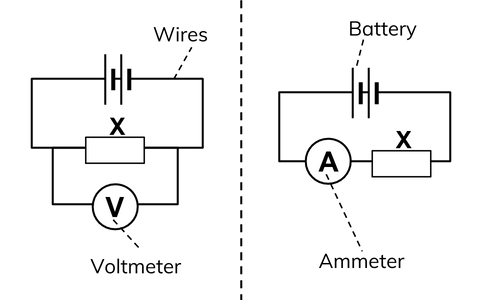Back

# Potential Difference

Potential difference is the difference in the amount of energy that charge carriers have between two points in a circuit.

**Measured in Volts: **Potential difference (p.d.) is measured in volts (V) and is also called voltage. The energy is transferred to the electrical components in a circuit when the charge carriers pass through them. We use a voltmeter to measure potential difference (or voltage).

Potential Difference formula:** V = I x R**

The potential difference (which is the same as voltage) is equal to the amount of current multiplied by the resistance. A potential difference of one Volt is equal to one Joule of energy being used by one Coulomb of charge when it flows between two points in a circuit.

## Measurements in Circuits

**Ammeters: **An ammeter measures the flow of current that passes through it. Ammeters have to be connected in series (in the same loop of the circuit) with the electrical component whose current you are measuring. For example component X above.

**Voltmeters: **Voltmeters measure the potential difference (voltage) between two points in a circuit. For example between two points either side of component X above. Voltmeters must always be connected in parallel (on a separate branch of the circuit) with the two points being measured.

**Current vs potential difference: **The current is a flow of charge. Current is measured through a component. Potential difference is the energy used between two points in a circuit, therefore it is measured between two points either side of a component. We describe this as the potential difference measured across a component.This diagram shows that an ammeter must be connected in series with the components you want to measure and a voltmeter must be connected in parallel.